# Volume per time

How long does fill take for a pump with a volume flow of 200 l per minute fill a cube-shaped tank up to 75% of its height if the length of the cube edge is 4 m?

t =  240 min

### Step-by-step explanation: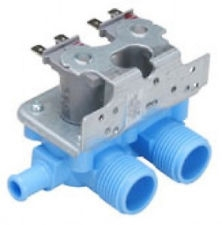Did you find an error or inaccuracy? Feel free to write us. Thank you!Tips to related online calculators
Do you know the volume and unit volume, and want to convert volume units?
Do you want to convert time units like minutes to seconds?

## Related math problems and questions:

• Water tank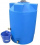The block-shaped tank is 2.5 m long, 100 cm wide and 12 dm high. In how many minutes will it be filled with water to two thirds if 40 liters of water flow into the tank per minute?
• Tank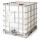How many minutes does it take to fill the tank to 25 cubic meters of water filled 150hl per hour?
• Water tank300hl of water was filled into the tank 12 m long and 6 m wide. How high does it reach?
• Cube basicsHow long is the edge length of a cube with volume 15 m3?
• The tankThe tank is full up to 4/5 of the total height and contains 240 hl of water. The area of the base is 6 square meters. What is the height of the tank?
• Granite cube statue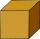What is the weight of a granite cube with an edge length of 75 cm, if 1 m3 of granite has a weight of 2.7 tons?
• Water level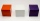How many cm will the water level in a cube-shaped tank with an edge of 3 m drop if we discharge 189 hl of water?
• The cubeThe cube has a surface area of 486 m ^ 2. Calculate its volume.
• Tank full2 hl of water were poured into a block-shaped tank with dimensions of 450 mm and 600 mm and a height of 900 mm. How many liters of water need to be added to keep the tank full?
• Cube-shaped containerThe cube-shaped container has a height of 52 cm and a square base. The container was filled to the brim with water, then we immersed a metal cube in it, which caused 2.7 l of water to flow out of the container. After removing the cube from the water, the
• Soil pit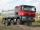Workers dug a pit cube with edge 2.5 meters. How many cars shall remove soil if the car suddenly take 4m³ of soil?
• Edges or sidesCalculate the cube volume, if the sum of the lengths of all sides is 276 cm.
• Water inlets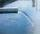Inlet valve with a flow rate of 12 liters per second is filled tank for 72 minutes. How long take to fill full tank if we open one more such valve half an hour after?
• Water tankWhat is the height of the cuboid-shaped tank with the bottom dimensions of 80 cm and 50 cm if the 480 liters of water reach 10 cm below the top?
• Pumps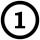First pump flows 16 liters per second into the basin by the second pump 75% of the first and by third pump half more than the second. How long will take fill basin by all three pumps simultaneously volume 15 m3 (cubic meters)?
• Cylindrical tank9.6 hl of water is poured into a cylindrical tank with a bottom diameter of 1.2 m. What height in centimeters does the water reach?
• TankTo cuboid tank whose bottom has dimensions of 9 m and 15 m were flow 1080 hectoliters of water. This was filled 40% of the tank volume. Calculate the depth of the tank.